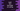# How to find the length of a string in swift## Introduction :

String in swift is a collection of characters. To find the length of a string, swift string comes with a count property. Read the count and you will get the total character count.

## Example of string.count :

Let’s take a look at the below example :

``````let string1 = "a"
let string2 = "a b"
let string3 = ""
let string4 = "!!"

print(string1.count)
print(string2.count)
print(string3.count)
print(string4.count)``````

It will print the below output :

``````1
3
0
2``````